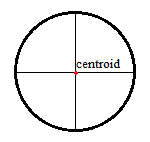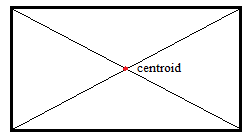# Centroid: Simple Definition, Examples, Finding

Contents:

## Definition & Basic Geometry

The centroid is the average of all points in an object. It is sometimes called the center of area or the center of volume.

## Uniform Density

If a shape has uniform density, the centroid is the same as the center of mass. If this shape is also in a uniform gravitational field, this is also the same as the center of gravity— the point where the whole weight of a body interacts. Exactly where the center is depends on the shape of the body; Surprisingly, the center of gravity doesn’t necessarily have to be inside the body. It might be a point outside, somewhere in space.

## Finding the Centroid of Plane Figures

There’s a hands-on way to find the centroid of a plane figure. Draw it on a piece of card, cut it out, and then find the place where it balances perfectly on a pin or on the tip of a pencil. In theory, this should be simple. But in practice, it takes a little skill to balance the shape. Plus, merely balancing the shape won’t give you information like where the coordinates of the shape are. Some centroids, like circles, rectangles and triangles, are even easier to find:

## 1. Circle

To find the center of the circle: fold the paper in half one way, then another:## 2. Rectangle

To find the center of the rectangle, fold the paper (diagonally) in half from corner to corner:## 3. Triangle

Find the place where the triangle’s three medians interact. To find any one median of a triangle, draw a line segment from one vertex (corner) to another point half way on the opposite side.## Finding the Centroid of Two Dimensional Shapes Using Calculus

Two methods can be used to find the centroid’s location:

## Summation

Let’s say we have a more complicated shape that we can turn into a compound shape (i.e. divide it into smaller primary shapes). If we know how to find the centroids for each of the individual shapes, we can find the compound shape’s centroid using the formula:Where:

• xi is the distance from the axis to the centroid of the simple shape,
• Ai is the area of the simple shape.
• Σ is summation notation, which basically means to “add them all up.”

The same formula, with yi substituting for xi, gives us the y coordinate of the centroid.

## Integration.

An integral can be used to find the centroid of shape too complicated to be broken down into its primary parts. Integrating is working with infinitesimally small areas; Finding the centroid of parts tell us what the centroid of the whole will be.

If a shape or region is bounded by two functions, f(x) and g(x), the coordinates of the centroid is given by:where A is the area calculated by the integral.

## References

Gere, J. & Goodno, B. (2008). Mechanics of Materials. Cengage Learning.
Pytel, A. & Kiusalaas, J. (2009). Engineering Mechanics: Statics. Cengage Learning.
Rajput, R. (2015). A Textbook of Applied Mechanics. Laxmi Publications.

CITE THIS AS:
Stephanie Glen. "Centroid: Simple Definition, Examples, Finding" From StatisticsHowTo.com: Elementary Statistics for the rest of us! https://www.statisticshowto.com/centroid/